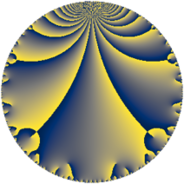# Properties

 Label 175.5.dLevel $175$ Weight $5$ Character orbit 175.d Rep. character $\chi_{175}(76,\cdot)$ Character field $\Q$ Dimension $47$ Newform subspaces $9$ Sturm bound $100$ Trace bound $2$

# Related objects

## Defining parameters

 Level: $$N$$ $$=$$ $$175 = 5^{2} \cdot 7$$ Weight: $$k$$ $$=$$ $$5$$ Character orbit: $$[\chi]$$ $$=$$ 175.d (of order $$2$$ and degree $$1$$) Character conductor: $$\operatorname{cond}(\chi)$$ $$=$$ $$7$$ Character field: $$\Q$$ Newform subspaces: $$9$$ Sturm bound: $$100$$ Trace bound: $$2$$ Distinguishing $$T_p$$: $$2$$

## Dimensions

The following table gives the dimensions of various subspaces of $$M_{5}(175, [\chi])$$.

Total New Old
Modular forms 86 53 33
Cusp forms 74 47 27
Eisenstein series 12 6 6

## Trace form

 $$47 q + 5 q^{2} + 341 q^{4} + q^{7} + 217 q^{8} - 1053 q^{9} + O(q^{10})$$ $$47 q + 5 q^{2} + 341 q^{4} + q^{7} + 217 q^{8} - 1053 q^{9} + 294 q^{11} - 491 q^{14} + 1725 q^{16} - 815 q^{18} - 60 q^{21} - 2058 q^{22} + 1490 q^{23} - 679 q^{28} + 14 q^{29} + 7977 q^{32} - 6219 q^{36} - 4270 q^{37} - 8700 q^{39} - 5580 q^{42} - 3610 q^{43} + 12592 q^{44} - 6992 q^{46} + 4447 q^{49} - 13620 q^{51} - 6178 q^{53} + 2123 q^{56} + 12900 q^{57} + 17262 q^{58} + 341 q^{63} + 579 q^{64} + 19150 q^{67} + 12834 q^{71} - 36703 q^{72} - 3232 q^{74} - 16810 q^{77} - 20100 q^{78} - 16786 q^{79} + 30447 q^{81} + 14280 q^{84} - 34072 q^{86} + 882 q^{88} + 48700 q^{91} + 49242 q^{92} + 70980 q^{93} - 60115 q^{98} - 27126 q^{99} + O(q^{100})$$

## Decomposition of $$S_{5}^{\mathrm{new}}(175, [\chi])$$ into newform subspaces

Label Dim $A$ Field CM Traces $q$-expansion
$a_{2}$ $a_{3}$ $a_{5}$ $a_{7}$
175.5.d.a $1$ $18.090$ $$\Q$$ $$\Q(\sqrt{-7})$$ $$-1$$ $$0$$ $$0$$ $$-49$$ $$q-q^{2}-15q^{4}-7^{2}q^{7}+31q^{8}+3^{4}q^{9}+\cdots$$
175.5.d.b $2$ $18.090$ $$\Q(\sqrt{21})$$ $$\Q(\sqrt{-7})$$ $$-1$$ $$0$$ $$0$$ $$98$$ $$q-\beta q^{2}+(31+\beta )q^{4}+7^{2}q^{7}+(-47+\cdots)q^{8}+\cdots$$
175.5.d.c $2$ $18.090$ $$\Q(\sqrt{-1})$$ $$\Q(\sqrt{-35})$$ $$0$$ $$0$$ $$0$$ $$0$$ $$q+17iq^{3}-2^{4}q^{4}+7^{2}iq^{7}-208q^{9}+\cdots$$
175.5.d.d $2$ $18.090$ $$\Q(\sqrt{21})$$ $$\Q(\sqrt{-7})$$ $$1$$ $$0$$ $$0$$ $$-98$$ $$q+\beta q^{2}+(31+\beta )q^{4}-7^{2}q^{7}+(47+2^{4}\beta )q^{8}+\cdots$$
175.5.d.e $4$ $18.090$ $$\Q(i, \sqrt{6})$$ None $$0$$ $$0$$ $$0$$ $$0$$ $$q-\beta _{2}q^{2}-\beta _{1}q^{3}-10q^{4}+\beta _{3}q^{6}+\cdots$$
175.5.d.f $8$ $18.090$ $$\mathbb{Q}[x]/(x^{8} + \cdots)$$ None $$-4$$ $$0$$ $$0$$ $$128$$ $$q+(-1-\beta _{2})q^{2}-\beta _{1}q^{3}+(1+\beta _{2}+\beta _{6}+\cdots)q^{4}+\cdots$$
175.5.d.g $8$ $18.090$ $$\mathbb{Q}[x]/(x^{8} - \cdots)$$ None $$0$$ $$0$$ $$0$$ $$0$$ $$q+\beta _{4}q^{2}+3\beta _{3}q^{3}+(22+\beta _{1})q^{4}-3\beta _{2}q^{6}+\cdots$$
175.5.d.h $8$ $18.090$ $$\mathbb{Q}[x]/(x^{8} + \cdots)$$ None $$4$$ $$0$$ $$0$$ $$-128$$ $$q+(1+\beta _{2})q^{2}-\beta _{1}q^{3}+(1+\beta _{2}+\beta _{6}+\cdots)q^{4}+\cdots$$
175.5.d.i $12$ $18.090$ $$\mathbb{Q}[x]/(x^{12} - \cdots)$$ None $$6$$ $$0$$ $$0$$ $$50$$ $$q-\beta _{1}q^{2}-\beta _{3}q^{3}+(10-\beta _{1}-\beta _{2})q^{4}+\cdots$$

## Decomposition of $$S_{5}^{\mathrm{old}}(175, [\chi])$$ into lower level spaces

$$S_{5}^{\mathrm{old}}(175, [\chi]) \cong$$ $$S_{5}^{\mathrm{new}}(7, [\chi])$$$$^{\oplus 3}$$$$\oplus$$$$S_{5}^{\mathrm{new}}(35, [\chi])$$$$^{\oplus 2}$$